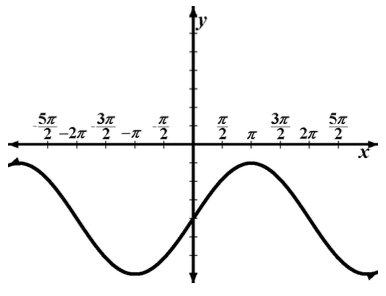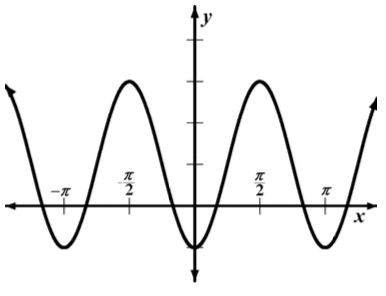### Home > PC > Chapter 7 > Lesson 7.1.1 > Problem7-13

7-13.

For each graph below, find two functions, one that uses sine and one that uses cosine, that will generate the graph. (Recall the 5-point method if you need help.)

1.The sine does not need a horizontal shift.
The cosine needs to be shifted $π$ to the left.

1.$\text{The sine needs to be shifted } \frac{\pi}{4} \text{ to the left.}$

The cosine needs to be flipped.